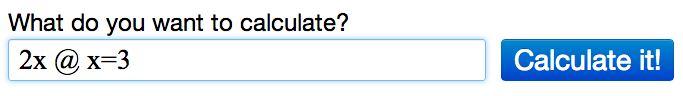# Evaluating Expressions Using Algebra Calculator

Learn how to use the Algebra Calculator to evaluate expressions.

### Example Problem

Evaluate the expression 2x for x=3.

### How to Evaluate the Expression in Algebra Calculator

First go to the Algebra Calculator main page.

Type the following:

1. First type the expression 2x.
2. Then type the @ symbol.
3. Then type x=3.
Try it now: 2x @ x=3

### Clickable Demo

Try entering 2x @ x=3 into the text box.After you enter the expression, Algebra Calculator will evaluate 2x for x=3: 2(3) = 6.### More Examples

Here are more examples of how to evaluate expressions in Algebra Calculator. Feel free to try them now.